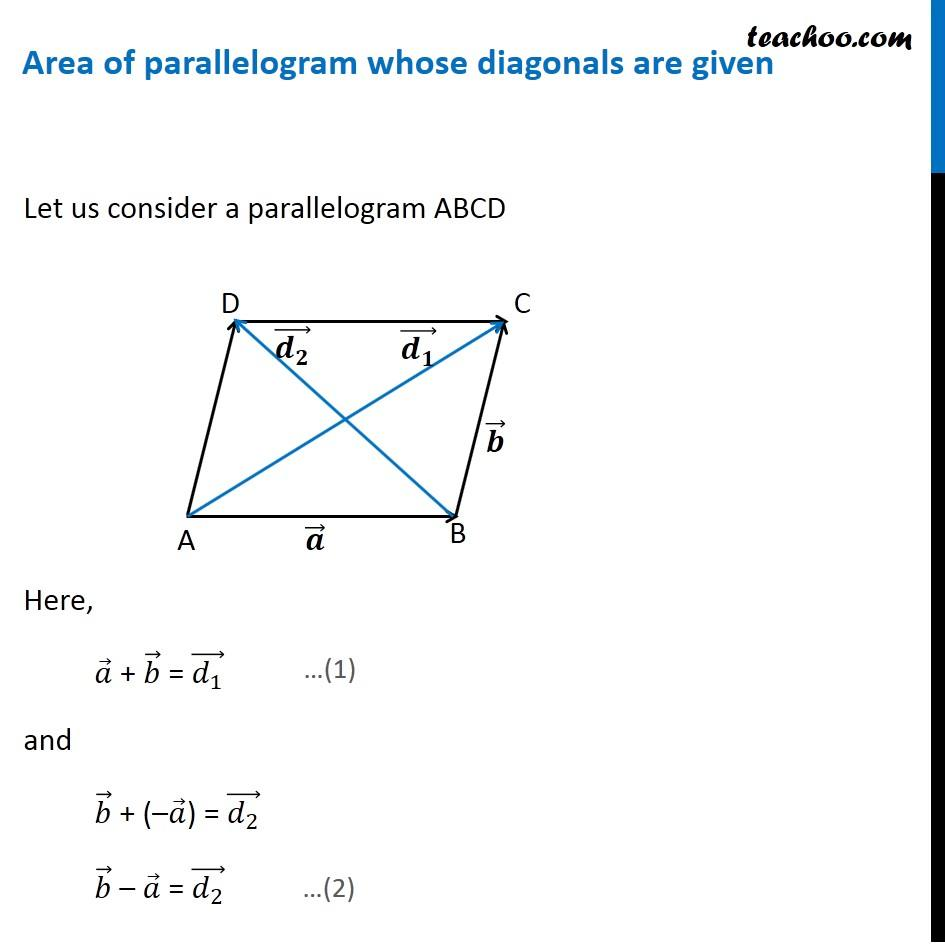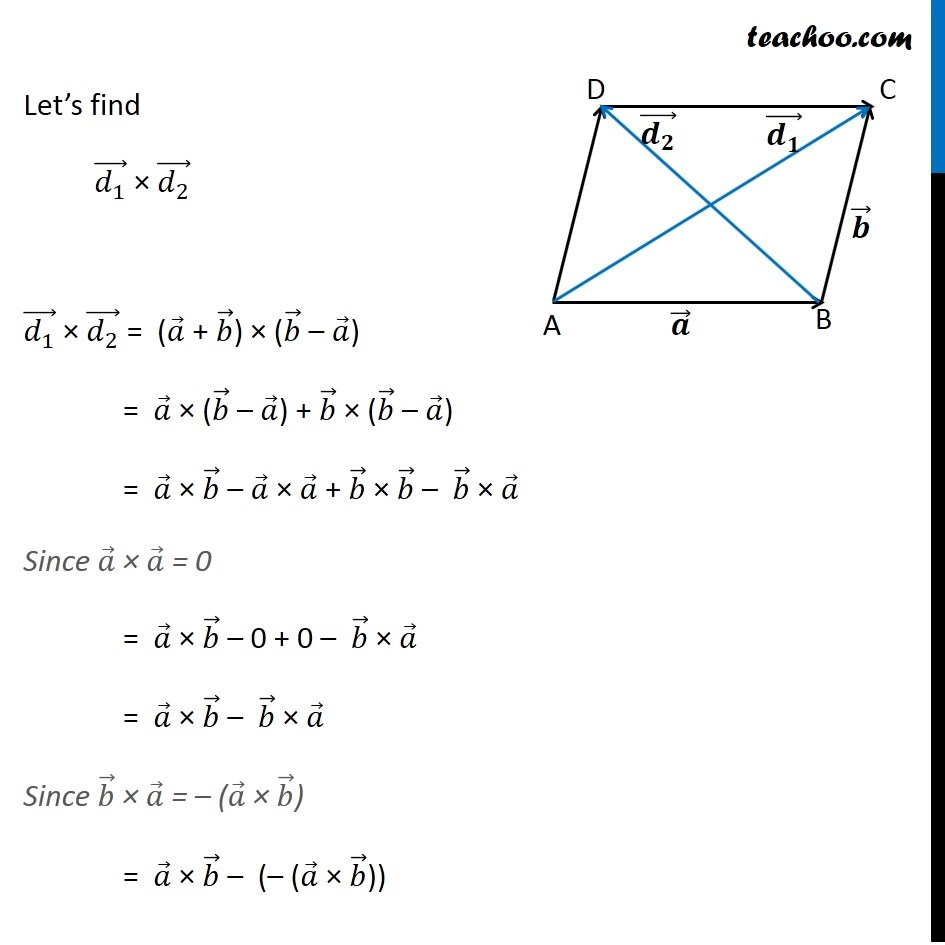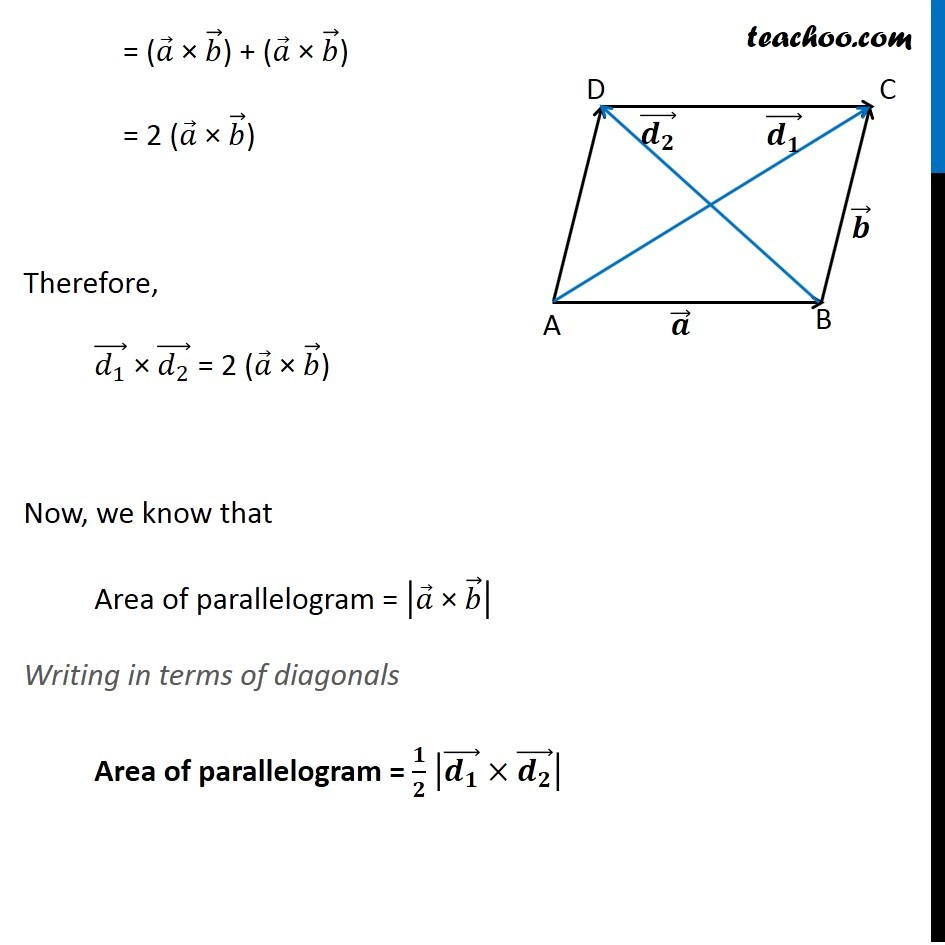Vector product - Area

Chapter 10 Class 12 Vector Algebra
Concept wiseCheck an Example Question here

Learn in your speed, with individual attention - Teachoo Maths 1-on-1 Class

### Transcript

Area of parallelogram whose diagonals are given Let us consider a parallelogram ABCD Here, 𝑎 ⃗ + 𝑏 ⃗ = (𝑑_1 ) ⃗ and 𝑏 ⃗ + (–𝑎 ⃗) = (𝑑_2 ) ⃗ 𝑏 ⃗ – 𝑎 ⃗ = (𝑑_2 ) ⃗ Let’s find (𝑑_1 ) ⃗ × (𝑑_2 ) ⃗ (𝑑_1 ) ⃗ × (𝑑_2 ) ⃗ = (𝑎 ⃗ + 𝑏 ⃗) × (𝑏 ⃗ – 𝑎 ⃗) = 𝑎 ⃗ × (𝑏 ⃗ – 𝑎 ⃗) + 𝑏 ⃗ × (𝑏 ⃗ – 𝑎 ⃗) = 𝑎 ⃗ × 𝑏 ⃗ – 𝑎 ⃗ × 𝑎 ⃗ + 𝑏 ⃗ × 𝑏 ⃗ – 𝑏 ⃗ × 𝑎 ⃗ Since 𝑎 ⃗ × 𝑎 ⃗ = 0 = 𝑎 ⃗ × 𝑏 ⃗ – 0 + 0 – 𝑏 ⃗ × 𝑎 ⃗ = 𝑎 ⃗ × 𝑏 ⃗ – 𝑏 ⃗ × 𝑎 ⃗ Since 𝑏 ⃗ × 𝑎 ⃗ = – (𝑎 ⃗ × 𝑏 ⃗) = 𝑎 ⃗ × 𝑏 ⃗ – (– (𝑎 ⃗ × 𝑏 ⃗)) = (𝑎 ⃗ × 𝑏 ⃗) + (𝑎 ⃗ × 𝑏 ⃗) = 2 (𝑎 ⃗ × 𝑏 ⃗) Therefore, (𝑑_1 ) ⃗ × (𝑑_2 ) ⃗ = 2 (𝑎 ⃗ × 𝑏 ⃗) Now, we know that Area of parallelogram = |𝑎 ⃗" × " 𝑏 ⃗ | Writing in terms of diagonals Area of parallelogram = 𝟏/𝟐 |(𝒅_𝟏 ) ⃗×(𝒅_𝟐 ) ⃗ |